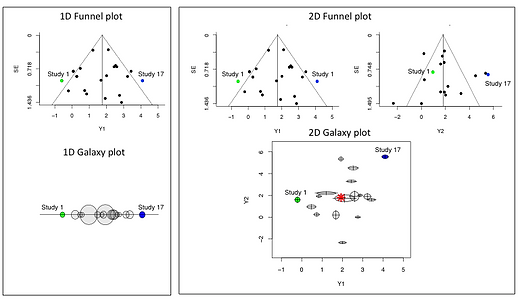top of page

## Tutorial 4.1: Galaxy plot in XMETA

The Galaxy plot provides a new method for displaying the information found in the funnel plot by creating one-dimensional and two-dimensional plots.

To aid in the visualization, we first reduce the dimension of a univariate funnel plot as demonstrated in the left panel of this figure. The main idea is to project the two-dimensional univariate funnel plot (i.e., effect size and standard error are displayed using two axes) to the one-dimensional galaxy plot. The one-dimensional Galaxy plot represents the effect size and standard error data points using circles on an axis. The axis is a continuum for effect size while the size of the circles on the axis corresponds with that study’s precision. Larger studies, and thus studies that are more precise, are represented by bigger circles. The size of each circle coincides with its contribution to the weighted sum estimate of the overall effect size. We can obtain a high-level view of where the effect sizes of different studies fall relative to each other through the one-dimensional galaxy plot .

The two-dimensional Galaxy plot incorporates a similar visualization technique and can be used to compare two outcomes. The x and y axes of the Galaxy plot are the two different outcomes being studied. Within the plane, each study is represented by an ellipse whose location and size are determined by the point estimates and precision (inverse of standard error) of the two outcomes. The crosshair in each ellipse helps to display the precision, and its intersection shows the estimate of the bivariate outcomes. The center of mass of the galaxy, which is marked by the red star among the ellipses, corresponds to the overall estimate of the two outcomes.

We term these as Galaxy plots for their similarity to images of galaxies.

## ​

(a). Galaxy-confidence plot:

The galaxy-confidence plot uses cross-hairs to represent the confidence intervals with the cross point showing the point estimate of the bivariate outcome. The confidence intervals are then compared with the lines representing no effect (i.e., black dash lines) to help determine the significance of the effect sizes.

(b). Galaxy-correlation plot:

The galaxy-correlation plot displays the within-study correlation of each individual study by an arrow starting from the center of the ellipse. We restrict the range of arrow to the right side of the ellipse, and the range of correlation (-1, 1) is mapped to the radian range (an arrow above the x-axis represents a positive correlation, while one below the x-axis represents a negative correlation).

(c). Galaxy-heterogeneity plot:

TThe galaxy-heterogeneity plot enables investigations of heterogeneity in a bivariate space. For example, the ellipses in green represent the studies comparing Treatment A and the placebo, while the ellipses in blue represent the studies comparing Treatment B and the placebo.

(d). PB investigation:

In the presence of PB, the galaxy plot is usually not symmetric around the estimated center of mass. More sophisticated statistical methods to quantify and correct for PB can be developed based on this Galaxy plot.Tutorial 5: Statistical Test to Evaluate Bias

bottom of page